Hello, I am back! After some summer rest/study/introspection! And after an amazing July month with the Higgs discovery by ATLAS and CMS. After an amazing August month with the Curiosity rover, MSL(Mars Science Laboratory), arrival to Mars. After a hot summer in my home town…I have written lots of drafts these days…And I will be publishing all of them step to step.

We will discuss today one of interesting remark studied by Kaniadakis. He is known by his works on relatistivic physics, condensed matter physics, and specially by his work on some cool function related to non-extensive thermodynamics. Indeed, Kaniadakis himself has probed that his entropy is also related to the mathematics of special relativity. Ultimately, his remarks suggest:

1st. Dimensionless quantities are the true fundamental objects in any theory.

2nd. A relationship between information theory and relativity.

3rd. The important role of deformation parameters and deformed calculus in contemporary Physics, and more and more in the future maybe.

4nd. Entropy cound be more fundamental than thought before, in the sense that non-extensive generalizations of entropy play a more significant role in Physics.

5th. Non-extensive entropies are more fundamental than the conventional entropy.

The fundamental object we are going to find is stuff related to the following function: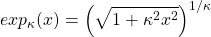Let me first imagine two identical particles ( of equal mass) A and B, whose velocities, momenta and energies are, in certain frame S: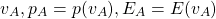In the rest frame of particle B, S’, we have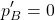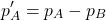If we define a dimensionless momentum paramenterwe get after usual exponentiation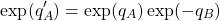Galilean relativity says that the laws of Mechanics are unchanged after the changes from rest to an uniform motion reference frame. Equivalentaly, galilean relativity in our context means the invariance under a change, and it implies the invariance under a change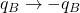. In turn, plugging these inte the last previous equation, we get the know relationship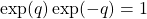Wonderful, isn’t it? It is for me! Now, we will move to Special Relativity. In the S’ frame where B is at rest, we have: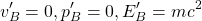and from the known relativistic transformations for energy and momentum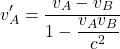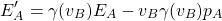where of course we define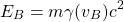After this introduction, we can parallel what we did for galilean relativity. We can write the last previous equations in the equivalent form, after some easy algebra, as follows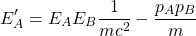Now, we can introduce dimensionless variables instead of the triple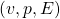, defining instead the adimensional set:Note that the so-called deformation parameter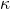is indeed related (equal) to the beta parameter in relativity. Again, from the special relativity requirementwe obtain, as we expected, that. Classical physics, the galilean relativity we know from our everyday experience, is recovered in the limit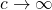, or equivalently, if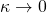. In the dimensionless variables, the transformation of energy and momentum we wrote above can be showed to be: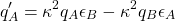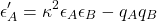In rest frame of some particle, we get of course the result, or in the new variables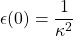. The energy-momentum dispersion relationship from special relativity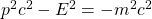becomes: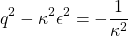or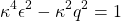Moreover, we can rewrite the equationin terms of the dimensionless energy-momentum variableand we get the analogue of the galilean addition rule for dimensionless velocitiesNote that the classical limit is recovered again sending. Now, we have to define some kind of deformed exponential function. Let us define: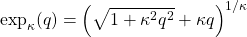Applying this function to the above last equation, we observe thatAgain, relativity means that observers in uniform motion with respect to each other should observe the same physical laws, and so, we should obtain invariant equations under the exchangesand. Pluggint these conditions into the last equation, it implies that the following condition holds (and it can easily be checked from the definition of the deformed exponential).One interesting question is what is the inverse of this deformed exponential ( the name q-exponential or-exponential is often found in the literature). It has to be some kind of deformed logarithm. And it is! The deformed logarithm, inverse to the deformed exponential, is the following function: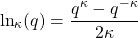Indeed, this function is related to ( in units with the Boltzmann’s constant set to the unit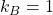) the so-called Kaniadakis entropy!Furthermore, the equation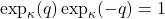also implies that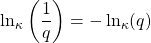The gamma parameter of special relativity is also recasted as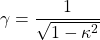More generally, in fact, the deformed exponentials and logarithms develop a complete calculus based on: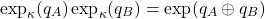and the differential operatorsso that, e.g.,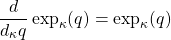This Kanadiakis formalism is useful, for instance, in generalizations of Statistical Mechanics. It is becoming a powertool in High Energy Physics. At low energy, classical statistical mechanics gets a Steffan-Boltmann exponential factor distribution function: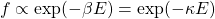At high energies, in the relativistic domain, Kaniadakis approach provide that the distribution function departures from the classical value to a power law:There are other approaches and entropies that could be interesting for additional deformations of special relativity. It is useful also in the foundations of Physics, in the Information Theory approach that sorrounds the subject in current times. And of course, it is full of incredibly beautiful mathematics!

We can start from deformed exponentials and logarithms in order to get the special theory of relativity (reversing the order in which I have introduced this topic here). Aren’t you surprised?

This site uses Akismet to reduce spam. Learn how your comment data is processed.